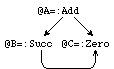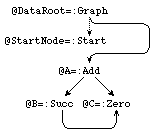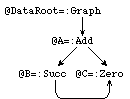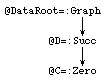Chapter 1
Basic Semantics

 1.1 Graph Rewriting

 1.2 Global Graphs

The semantics of Clean is based on Term Graph Rewriting Sys­tems (Barendregt, 1987; Plas­me­i­jer and Van Eekelen, 1993). This means that functions in a Clean pro­gram se­mantically work on graphs in­stead of the usual terms. This enabled us to incorporate Clean’s typical features (definition of cyclic data structures, lazy copying, uniqueness typing) which would otherwise be very difficult to give a pro­per semantics for. However, in many cases the programmer does not need to be aware of the fact that he/she is manipulating graphs. Evaluation of a Clean program takes place in the same way as in other lazy functional languages. One of the “differ­ences” between Clean and other functional languages is that when a variable occurs more than once in a function body, the se­mantics pre­scribe that the actual argument is shared (the semantics of most other lan­guages do not pre­s­cribe this although it is common practice in any implementation of a func­tional lan­guage). Fur­ther­more, one can label any expression to make the definition of cyclic structures possible. So, people familiar with other functio­nal languages will have no problems writing Clean programs.

When larger applications are being written, or, when Clean is interfaced with the non-functio­nal world, or, when efficiency counts, or, when one simply wants to have a good understanding of the lan­guage it is good to have some knowledge of the basic semantics of Clean which is based on term graph rewriting. In this chapter a short introduction into the basic semantics of Clean is given. An ex­tensive treatment of the underlying semantics and the implementation techniques of Clean can be found in Plasmeijer and Van Eekelen (1993).

## 1.1    Graph Rewriting

A Clean program basically consists of a number of graph rewrite rules (function definitions) which spe­c­ify how a given graph (the initial expression) has to be rewritten

A graph is a set of nodes. Each node has a defining node-identifier (the node-id). A node con­sists of a sym­bol and a (possibly empty) sequence of applied node-id’s (the argu­ments of the symbol)  Applied node-id’s can be seen as refer­ences (arcs) to nodes in the graph, as such they have a direc­tion: from the node in which the node-id is applied to the node of which the node-id is the defining identifier.

Each graph rewrite rule consists of a left-hand side graph (the pattern) and a right-hand side (rhs) consist­ing of a graph (the contractum) or just a single node-id (a redirec­tion). In Clean rewrite rules are not comparing: the left-hand side (lhs) graph of a rule is a tree, i.e. each node identi­fier is applied only once, so there exists ex­actly one path from the root to a node of this graph.

A rewrite rule defines a (partial) function The function symbol is the root symbol of the left-hand side graph of the rule alternatives. All other symbols that appear in rewrite rules, are con­structor symbols.

The program graph is the graph that is rewritten according to the rules. Initially, this pro­gram graph is fixed: it con­sists of a single node containing the symbol Start, so there is no need to spec­ify this graph in the program ex­plic­itly. The part of the graph that matches the pat­tern of a cer­tain rewrite rule is cal­led a redex (reducible ex­pression). A rewrite of a redex to its reduct can take place ac­cording to the right-hand side of the cor­respond­ing rewrite rule. If the right-hand side is a contrac­tum then the rewrite consists of building this contrac­tum and doing a redi­rec­tion of the root of the re­dex to root of the right-hand side. Otherwise, only a redirec­tion of the root of the re­dex to the single node-id specified on the right-hand side is per­formed. A redirection of a node-id n1 to a node-id n2 means that all ap­plied occur­rences of n1 are replaced by occurrences of n2 (which is in re­ality com­monly imple­mented by overwriting n1 with n2).

A reduction strategy is a function that makes choices out of the available redexes. A re­du­cer is a pro­cess that reduces redexes that are indicated by the strategy. The result of a reducer is rea­ched as soon as the re­duction strategy does not indicate redexes any more. A graph is in nor­mal form if none of the patterns in the rules match any part of the graph. A graph is said to be in root normal form when the root of a graph is not the root of a redex and can never become the root of a redex. In general it is undecidable whether a graph is in root normal form.

A pattern partially matches a graph if firstly the symbol of the root of the pattern equals the sym­bol of the root of the graph and sec­ondly in positions where symbols in the pattern are not syn­tac­ti­cally equal to symbols in the graph, the corresponding sub-graph is a redex or the sub-graph it­self is par­tially matching a rule. A graph is in strong root normal form if the graph does not par­tially match any rule. It is decidable whether or not a graph is in strong root normal form. A graph in strong root nor­mal form does not partially match any rule, so it is also in root nor­mal form.

The default reduction strategy used in Clean is the functional reduction strategy. Redu­c­ing graphs ac­cor­ding to this strategy resembles very much the way exe­cution proceeds in other lazy func­tional lan­gua­ges: in the standard lambda calculus semantics the functional strategy corre­s­ponds to nor­mal order re­duction. On graph rewrite rules the functional strategy proceeds as fol­lows: if there are sev­eral rewrite rules for a par­ticular function, the rules are tried in textual or­der; pat­terns are tested from left to right; evaluation to strong root normal form of ar­guments is forced when an ac­tual ar­gu­ment is matched against a corresponding non-variable part of the pat­tern. A formal def­ini­tion of this strategy can be found in (Toyama et al., 1991).

### 1.1.1 A Small Example

Consider the following Clean program:

Add Zero z       =    z                         (1)

Start            =    Add (Succ o) o

where

o = Zero              (3)

In Clean a distinction is between function definitions (graph rewriting rules) and graphs (constant de­f­ini­tions). A semantic equivalent definition of the program above is given below where this dis­tinction is made explicit (“=>” indi­cates a rewrite rule whereas "=:" is used for a constant (sub-) graph def­inition

Add Zero z       =>    z                        (1)

Start            =>    Add (Succ o) o

where

o =: Zero            (3)

These rules are internally translated to a semantically equivalent set of rules in which the graph struc­ture on both left-hand side as right-hand side of the rewrite rules has been made explicit by adding node-id’s. Using the set of rules with explicit node-id’s it will be easier to understand what the mean­ing is of the rules in the graph rewriting world.

y =: Zero        =>   z                         (1)

y =: Succ a      =>   m =: Succ n

n =: Add a z              (2)

x =: Start       =>   m =: Add n o

n =: Succ o

o =: Zero                 (3)

The fixed initial program graph that is in memory when a program starts is the follow­ing:

 The initial graph in linear notation:   @DataRoot     =: Graph @StartNode @StartNode    =: Start The initial graph in pictorial notation:To distinguish the node-id’s appearing in the rewrite rules from the node-id’s appearing in the graph the lat­ter al­ways begin with a ‘@’.

The initial graph is rewritten until it is in normal form. Therefore a Clean program must at least con­tain a “ start rule” that matches this initial graph via a pat­tern. The right-hand side of the start rule spe­c­i­fies the actual computa­tion. In this start rule in the left-hand side the symbol Start is used. However, the symbols Graph and Initial (see next Section) are internal, so they cannot ac­tu­ally be addressed in any rule.

The patterns in rewrite rules contain formal node-id’s. During the matching these formal nodei­d’s are mapped to the ac­tual node-id’s of the graph  After that the following se­mantic actions are per­formed:

The start node is the only redex matching rule (3). The contractum can now be con­structed:

 The contractum in linear notation:   @A =: Add  @B @C @B =: Succ @C @C =: Zero The contractum in pictorial notation:All applied occurrences of @StartNode will be replaced by occurrences of @A. The graph after re­writ­ing is then:

 The graph after rewriting:   @DataRoot     =: Graph @A @StartNode    =: Start @A =: Add  @B @C @B =: Succ @C @C =: Zero Pictorial notation:This completes one rewrite. All nodes that are not accessible from @DataRoot are garbage and not con­si­dered any more in the next rewrite steps. In an implementation once in a while garbage col­lec­tion is per­formed in order to re­claim the memory space occupied by these garbage nodes. In this ex­ample the start node is not accessible from the data root node after the rewrite step and can be left out.

 The graph after garbage collection:   @DataRoot     =: Graph @A @A =: Add  @B @C @B =: Succ @C @C =: Zero Pictorial notation :The graph accessible from @DataRoot still contains a redex. It matches rule 2 yielding the ex­pected nor­mal form:

 The final graph:   @DataRoot =: Graph @D @D =: Succ @C @C =: Zero Pictorial notation :The fact that graphs are being used in Clean gives the programmer the abil­ity to explicitly share terms or to create cyclic structures. In this way time and space ef­ficiency can be obtained.

## 1.2    Global Graphs

Due to the presence of global graphs in Clean the initial graph in a specific Clean program is slightly different from the basic semantics. In a specific Clean program the initial graph is defined as:

@DataRoot    =: Graph @StartNode @GlobId1 @GlobId2 … @GlobIdn

@StartNode   =: Start

@GlobId1     =: Initial

@GlobId2     =: Initial

@GlobIdn     =: Initial

The root of the initial graph will not only contain the node-id of the start node, the root of the graph to be rewritten, but it will also contain for each global graph (see 10.2) a reference to an initial node (initialized with the symbol Initial). All references to a specific global graph will be references to its initial node or, when it is rewritten, they will be references to its reduct.